# Common Core: 1st Grade Math : Number & Operations in Base Ten

## Example Questions

← Previous 1 3 4 5 6 7 8 9 37 38

### Example Question #1 : Count, Read, And Write Numbers 1 120: Ccss.Math.Content.1.Nbt.A.1

What number is one hundred twenty?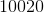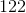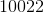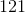Explanation:

In number form, one hundred twenty is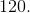### Example Question #1 : Count, Read, And Write Numbers 1 120: Ccss.Math.Content.1.Nbt.A.1

What number is ninety-nine?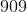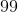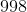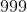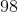Explanation:

In number form, ninety-nine is.

### Example Question #1 : Extend The Counting Sequence

What number is twenty-three?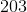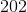Explanation:

In number form, twenty-three is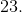### Example Question #1 : Count, Read, And Write Numbers 1 120: Ccss.Math.Content.1.Nbt.A.1

What number is forty-two?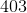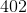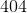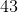Explanation:

In number form, forty-two is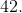### Example Question #1 : Extend The Counting Sequence

What number is nineteen?Explanation:

In number form, nineteen is.

### Example Question #2 : Extend The Counting Sequence

What number is one hundred thirteen?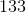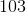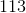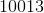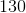Explanation:

In number form, one hundred thirteen is.

### Example Question #1 : Count, Read, And Write Numbers 1 120: Ccss.Math.Content.1.Nbt.A.1

What number is fifty-seven?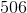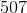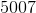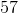Explanation:

In number form, fifty-seven is.

### Example Question #8 : Count, Read, And Write Numbers 1 120: Ccss.Math.Content.1.Nbt.A.1

What number is seventy-eight?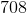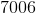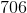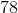Explanation:

In number form, seventy-eight is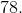### Example Question #3 : Extend The Counting Sequence

What number is eighty-nine?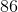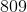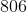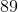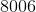Explanation:

In number form, eighty-nine is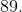### Example Question #2 : Count, Read, And Write Numbers 1 120: Ccss.Math.Content.1.Nbt.A.1

What number is one hundred eleven?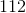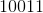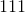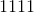In number form, one hundred eleven is.